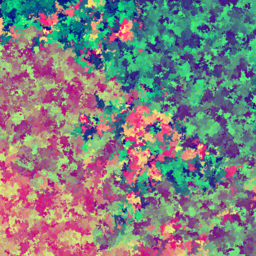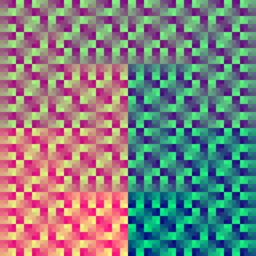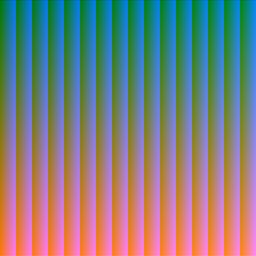AllRGB is a really cool challenge: make an image that contains each of the 16777216 RGB colors exactly once.

I wrote a HTML canvas-based visualization of an AllRGB image being created. It uses several space-filling curves in 2D and 3D.

I made a demo of this at /projects/allrgb and the source is on GitHub.

# Mapping cubes and planes to a line

My goal: traverse the RGB cube and also traverse the square pixel plane in an interesting way. In other words, create a list of 2563 unique 3D coordinates (representing 24-bit RGB colors) and a list of 40962 unique 2D coordinates (representing pixel locations).

I chose these traversal algorithms:

• Cube traversal
• Depth-first search (3D DFS)
• Serpentine (3D)
• Plane traversal
• Moore curve (a variant of the Hilbert curve)
• Depth-first search (2D DFS)
• Serpentine (2D)

## Example: Serpentine

Serpentine traversal is the simplest traversal algorithm I had. Note that it is not a space filling 'curve': it jumps after finishing a row, so it's not continuous.

Pseudocode for plane traversal

``````y = 0
while y < 4096:
x = 0
while x < 4096:
print (x, y)
x = x + 1
y = y + 1
``````

RGB cube traversal is a bit more interesting. While this can be implemented this as three nested loops, an easier solution is to convert the input number to hexadecimal.

``````// converts an integer n from [0..16777215] into the corresponding hex color (#RRGGBB)
function getColor(n) {
}
``````

## Example: Moore curveStages 0 through 5 of fractal Moore curve.By Robert Dickau [CC BY-SA 3.0], via Wikimedia Commons

This was the most interesting traversal algorithm I did. The Moore curve is a space-filling fractal, the loop variant of the Hilbert curve.

It can be represented as a Lindenmayer rewrite system:

• Variables: `L`, `R`
• Constants: `F`, `+`, `−`
• Axiom: `LFL+F+LFL`
• Production rules:
• `L``−RF+LFL+FR−`
• `R``+LF−RFR−FL+`

If you apply these rules recursively to the axiom, you get a set of instructions for moving a turtle. The instructions are string of constants where each character has the following meaning:

• `F`: draw at current location and move forward in current direction
• `+`: turn right 90°
• `-`: turn left 90°

This is a recursive function to generate L-system instructions:

``````function generateInstructions(level) {
if (level === 0) { // base case
// return axiom to start the instructions
return "LFL+F+LFL";
}

// recursive case
let instructions = generateInstructions(level - 1);

// replace 'L's and 'R's with their respective production rules
// 'X' is a temporary variable representing the original Ls
return instructions.replace(/L/g, "X")
.replace(/R/g, "+LF-RFR-FL+")
.replace(/X/g, "-RF+LFL+FR-");
}
``````

After cleaning up the final instructions, you get this:

``````> // clean up the instructions by replacing useless parts like +-, -+, R, and L
> generateInstructions(3).replace(/(\-\+)|(\+\-)|R|L/g, ""))
"-F+F+F-FF-F-F+F+F-F-FF-F+F+FF+F-F-F+FF+F+F-F-F+F+FF+F-F-FFF-F-F+FF+F+F-F-F+F+FF+
F-F-F+FF+F+F-FF-F-F+F+F-F-FF-F+F+F-F-F+F+F-FF-F-F+F+F-F-FF-F+F+FF+F-F-F+FF+F+F-F-
F+F+FF+F-F-FFF-F-F+FF+F+F-F-F+F+FF+F-F-F+FF+F+F-FF-F-F+F+F-F-FF-F+F+FFF+F+F-FF-F
-F+F+F-F-FF-F+F+FF+F-F-F+FF+F+F-F-F+F+FF+F-F-FFF-F-F+FF+F+F-F-F+F+FF+F-F-F+FF+F+
F-FF-F-F+F+F-F-FF-F+F+F-F-F+F+F-FF-F-F+F+F-F-FF-F+F+FF+F-F-F+FF+F+F-F-F+F+FF+F-F
-FFF-F-F+FF+F+F-F-F+F+FF+F-F-F+FF+F+F-FF-F-F+F+F-F-FF-F+F+F-"
``````

To traverse the full pixel plane, I needed an instruction set with exactly 40962 = 16777216 'draw' instructions. (There is an implicit `F` at the beginning of the instruction set, so I actually needed 16777215 `F`s.) The following table shows that I needed to recurse 11 times for this.

Recursion Levelinstructions.lengthNum of `F`s
093
14915
220963
3849255
434091023
5136494095
65460916383
721844965535
8873809262143
934952491048575
10139810094194303
115592404916777215

# Strategy design pattern

This was intended to be a quick project, so I would have normally made each of the traversers into a function in `script.js`, but I wanted to organize my code better. It made the most sense to separate out the code for the traversers, so I decided to use the strategy design pattern.

Each of the traversers (both cube and plane) has a very similar interface. They need a function to return their name and another to return the next traversed value.

Strategy design pattern code:

``````// Interface for Traverser strategy
class Traverser {
constructor(LEVEL, CANVAS_GRID_SIZE) {/* */}
getName() {/* */}
*generator() {/* */}
}
``````
``````// Maps names to strategies
const traversers = {
plane: {
'serpentine': SerpentinePlane,
'moore': MoorePlane,
'dfs': DepthFirstSearchPlane,
},
cube: {
'serpentine': SerpentineCube,
'dfs': DepthFirstSearchCube,
'hsl': HslCube,
}
};
// ...
// Creates new objects, representing traversal strategies
const planeTraverser = new traversers.plane['moore'](level, gridSize);
const cubeTraverser = new traversers.cube['dfs'](level, gridSize);
``````

# JavaScript generators

I initially implemented all my traversers as plain functions, but that had a lot of messy recalculation and non-local variables. Then I learned about a fancy newish feature in JavaScript called generators. Generators are well supported in modern browsers, so I felt safe using them in my project.

Using generators made my code more memory-efficient and cleaner.

Example of plane traverser using a generator:

``````// Generator that returns an RGB color
function* serpentine() {
for (let n = 0; n < 16777216; n++) {
}
}
// ...
let planeTraverser = serpentine();
planeTraverser.next(); // {value: "#000000", done: false}
planeTraverser.next(); // {value: "#000001", done: false}
``````

# Results!

Moore plane + DFS cube is my favorite.

DFS planeMoore planeSerpentine plane
DFS cube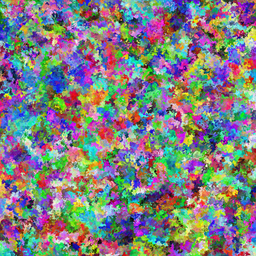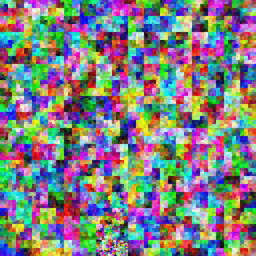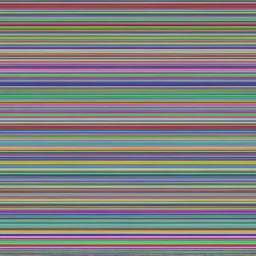Serpentine cube